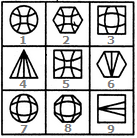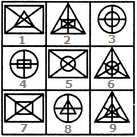# Non Verbal Reasoning - Grouping of Images

### Exercise :: Grouping of Images - Section 1

In each of the following questions, group the given figures into three classes using each figure only once.

6.

Group the given figures into three classes using each figure only once.A. 1,5,7 ; 2,4,6 ; 3,9,8 B. 1,5,7 ; 2,4,8 ; 3,6,9 C. 1,4,7 ; 2,5,8 ; 3,6,9 D. 1,7,9 ; 3,5,8 ; 2,4,6

Explanation:

1, 5, 7 have two similar elements, one inside the other.

2, 4, 8 have one element placed inside a different element.

3, 6, 9 have two similar elements, one inside the other and the area between the two elements is shaded.

7.

Group the given figures into three classes using each figure only once.A. 1,2,6 ; 3,4,7 ; 5 B. 1,3 ; 2,6 ; 4,5,7 C. 1,2,6,7 ; 3 ; 4,5 D. 1,3 ; 2,4,5 ; 6,7

Explanation:

1, 3 contain a V-shaped element inside a geometrical figure.

2, 4, 5 contain two similar elements, one placed inside the other and touching it.

6, 7 contain geometrical figures which are divided into four equal parts by two mutually perpendicular straight lines.

8.

Group the given figures into three classes using each figure only once.A. 1,2,5 ; 3,7,8 ; 4,6,9 B. 1,7,2 ; 3,9,6 ; 4,5,8 C. 2,3,8 ; 4,6,9 ; 1,5,7 D. 5,6,9 ; 3,4,1 ; 2,7,8

Explanation:

1, 2, 5 are figures that have patterns formed from four lines curved in a concave direction.

3, 7, 8 are figures that have patterns formed from four lines curved in a convex direction.

4, 6, 9 are figures that have patterns formed from these straight lines.

9.

Group the given figures into three classes using each figure only once.A. 2,4,7 ; 1,8,9 ; 3,5,6 B. 2,6,9 ; 1,5,7 ; 3,4,8 C. 2,6,7 ; 1,5,8 ; 3,4,9 D. 2,8,7 ; 1,5,9 ; 3,4,6

Explanation:

1, 5, 7 contain a rectangle with its two diagonals as the outer element and another element (similar or different) placed inside it.

2, 6, 9 contain a triangle with its three medians as the outer element and another element (similar or different) placed inside it.

3, 4, 8 contain a circle with its two mutually perpendicular diameters as the outer element and another element (similar or different) placed inside it.

10.

Group the given figures into three classes using each figure only once.A. 1,3,5 ; 2,6,9 ; 4,7,8 B. 2,3,4 ; 5,6,8 ; 9,1,7 C. 1,3,5 ; 2,6,8 ; 4,7,9 D. 3,2,4 ; 6,5,8 ; 7,9,1

Explanation:

1, 3, 5 are figures having partially or completely curved boundaries.

2, 6, 8 are all triangles.

4, 7, 9 are all quadrilaterals.